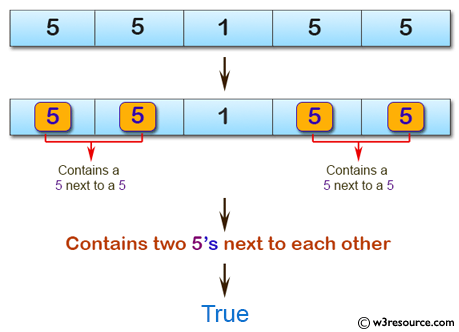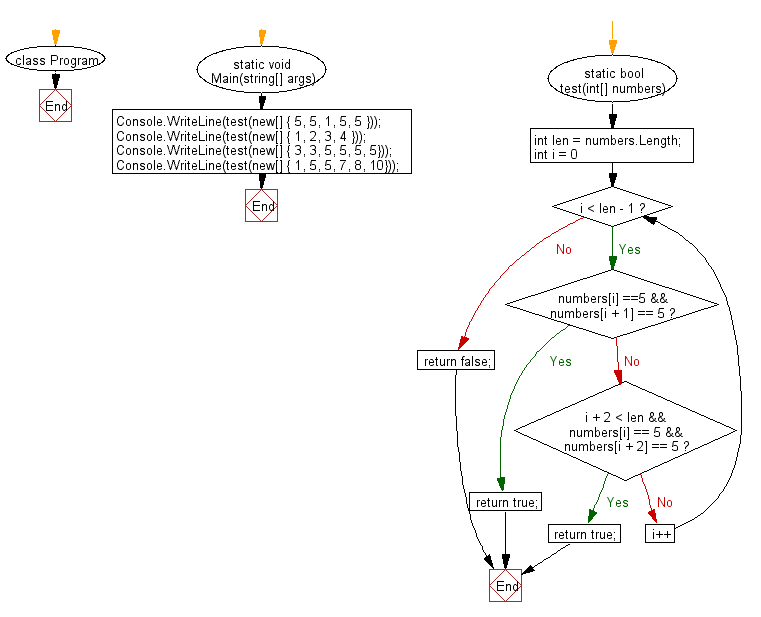﻿ C# - A couple of 5s next to each other or separated by one# C# Sharp Basic Algorithm Exercises: Check a given array of integers and return true if the given array contains two 5's next to each other, or two 5 separated by one element

## C# Sharp Basic Algorithm: Exercise-120 with Solution

Write a C# Sharp program to check a given array of integers and return true if the given array contains two 5's next to each other, or two 5 separated by one element.

Pictorial Presentation:Sample Solution:

C# Sharp Code:

``````using System;
namespace exercises
{
class Program
{
static void Main(string[] args)
{
Console.WriteLine(test(new[] { 5, 5, 1, 5, 5 }));
Console.WriteLine(test(new[] { 1, 2, 3, 4 }));
Console.WriteLine(test(new[] { 3, 3, 5, 5, 5, 5}));
Console.WriteLine(test(new[] { 1, 5, 5, 7, 8, 10}));
}
static bool test(int[] numbers)
{
int len = numbers.Length;

for (int i = 0; i < len - 1; i++)
{
if (numbers[i] ==5 && numbers[i + 1] == 5) return true;
if (i + 2 < len && numbers[i] == 5 && numbers[i + 2] == 5) return true;
}

return false;
}
}
}
```
```

Sample Output:

```True
False
True
True
```

Flowchart:C# Sharp Code Editor:

Improve this sample solution and post your code through Disqus

What is the difficulty level of this exercise?

Test your Programming skills with w3resource's quiz.

﻿